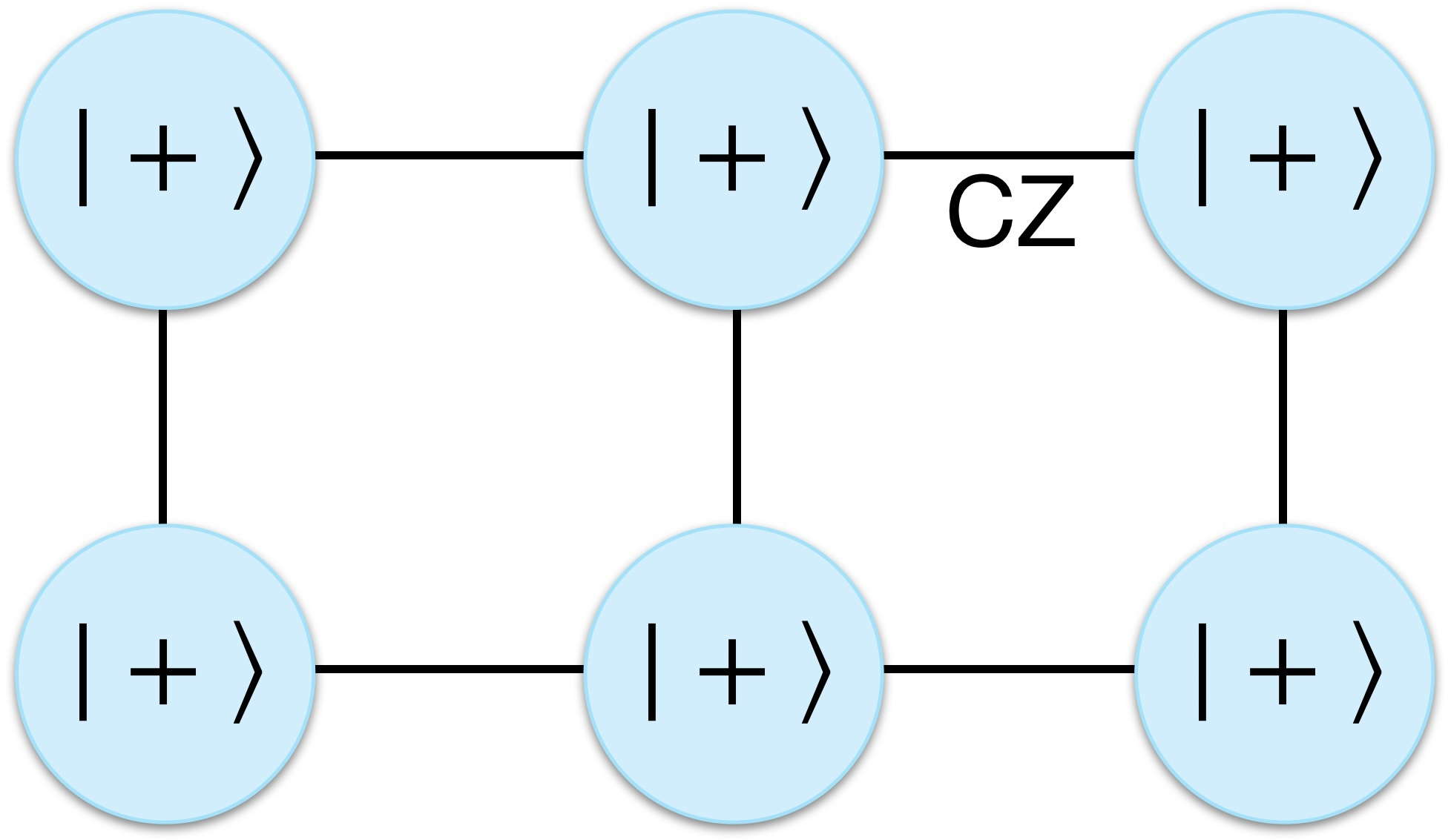# An introduction to graph states

#### What is a graph state?

One especially useful class of quantum states is graph states, also known as cluster states. As the name suggests, a graph state |G\rangle is associated with a graph,

G=(V,E),

where the vertices (v\in V) represent qubits initialised into the

|+\rangle = (|0\rangle+|1\rangle)/\sqrt{2}

state, and edges (e\in E) represent the application of controlled-phase (CZ) gates between respective vertices, where,

\mathrm{CZ}=\mathrm{diag}(1,1,1,-1).

A graph state can therefore be expressed as,

|G\rangle = \left[ \prod_{e\in E} \mathrm{CZ}_e \right] |+\rangle^{\otimes |V|}.

Since CZ gates are diagonal and therefore commute with one another, the order in which they are applied is irrelevant, meaning there is great flexibility in the preparation of graph states and room for parallelisation in the application of the required CZ gates.A graph state is defined with respect to a graph, where vertices represent qubits initialised into the |+\rangle state and edges represent the application of CZ gates between qubits. Since CZ gates commute, the order in which they are applied is irrelevant.
Continue reading An introduction to graph states